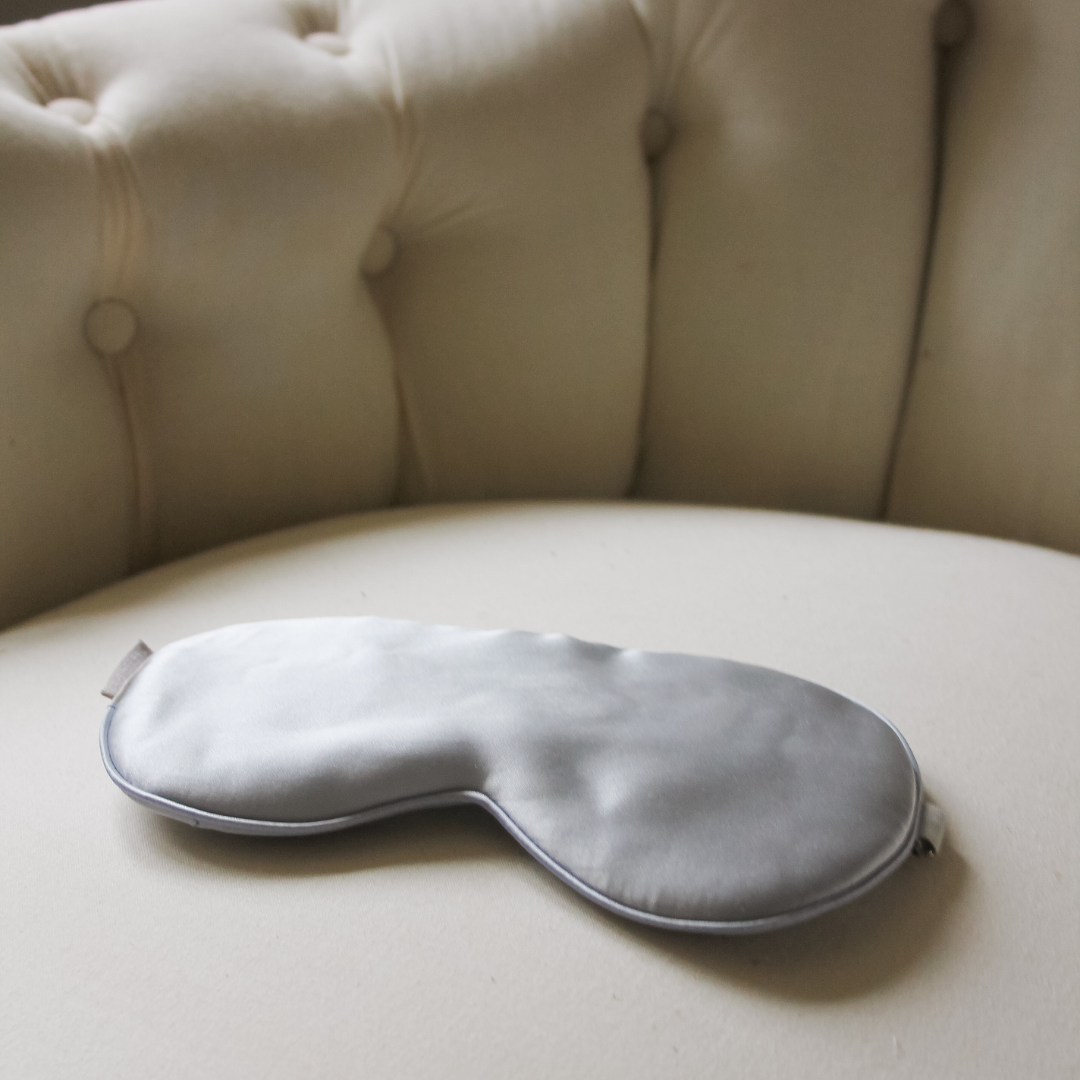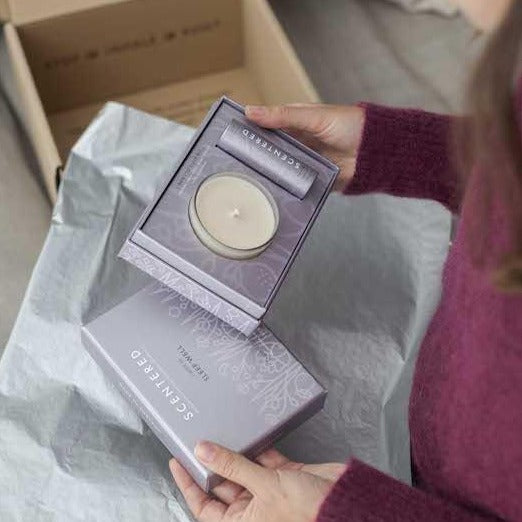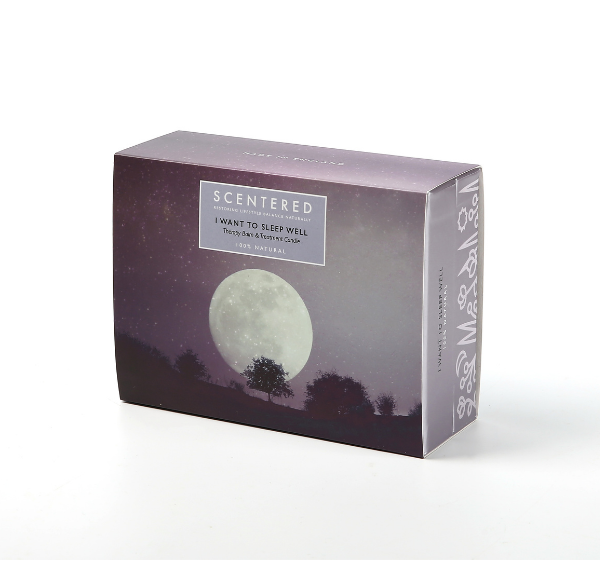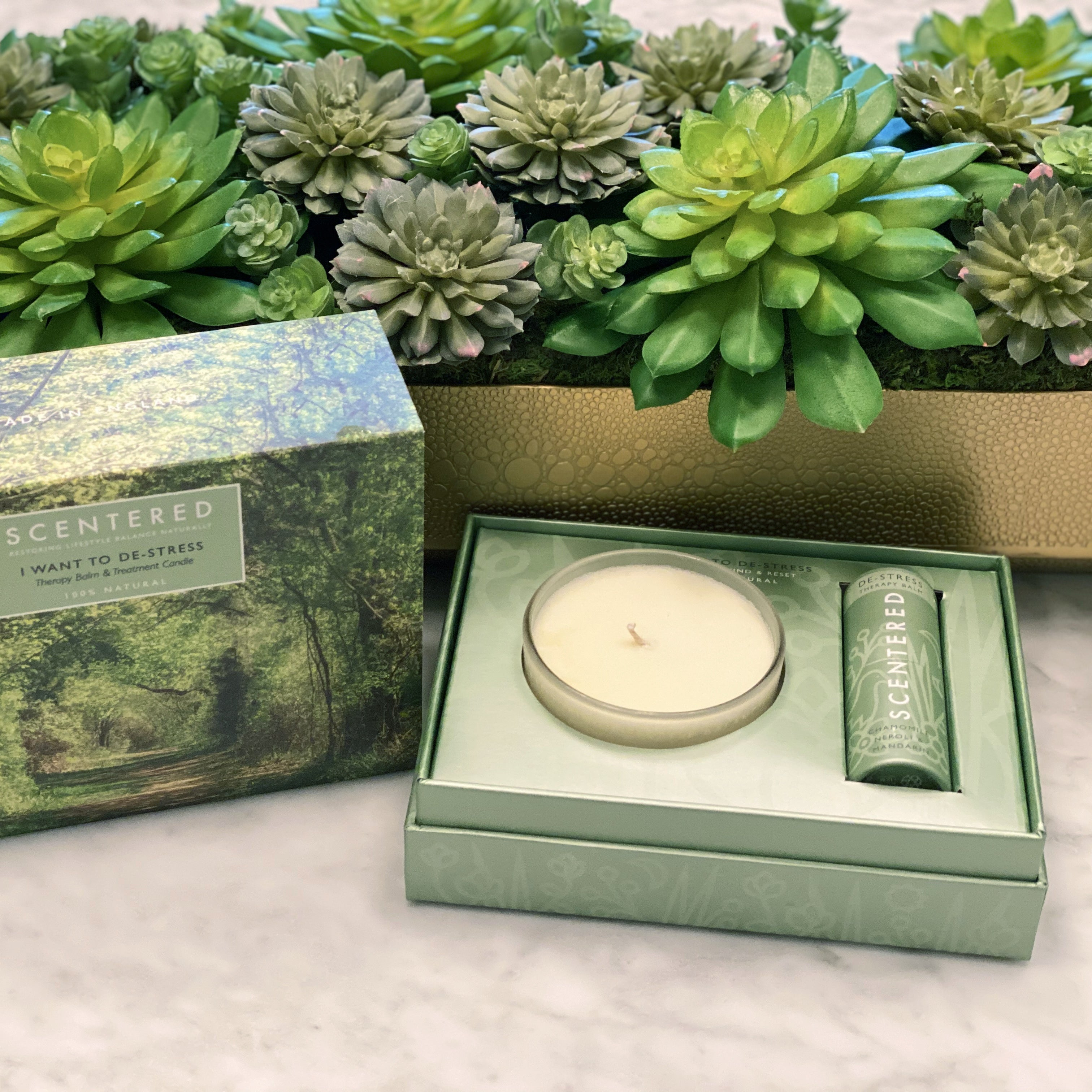# Everything except Mini balms, weighted blanket, Luxury SW set, Wellbei### S\$i\$l\$k\$ E\$y\$

£19.99### S\$L\$E\$E\$P\$ W\$E\$L\$L\$ W\$e\$l\$l\$b\$e\$i\$n\$g\$ R\$i\$t\$

De £16.00### D\$E\$-S\$T\$R\$E\$S\$S\$ W\$e\$l\$l\$b\$e\$i\$n\$g\$ R\$i\$t\$u

De £16.00### H\$A\$P\$P\$Y\$ W\$e\$l\$l\$b\$e\$i\$n\$g\$ R\$i\$t\$u\$a\$

De £16.90### I\$ W\$a\$n\$t\$ T\$o\$ S\$L\$E\$E\$P\$ W

£29.50### L\$O\$V\$E\$ W\$e\$l\$l\$b\$e\$i\$n\$g\$ R\$i\$t\$u\$a\$l

De £16.90### E\$S\$C\$A\$P\$E\$ W\$e\$l\$l\$b\$e\$i\$n\$g\$ R\$i\$t\$u\$a

De £16.90### D\$E\$-S\$T\$R\$E\$S\$S\$ H\$o\$m\$e\$ A\$r\$o\$m

De £16.95### I\$ W\$a\$n\$t\$ T\$o\$ D\$E\$-S\$T\$R\$

£29.50### E\$S\$C\$A\$P\$E\$ H\$o\$m\$e\$ A\$r\$o\$m\$a

De £16.95### D\$a\$i\$l\$y\$ R\$i\$t\$u\$a\$l\$

£38.90### F\$O\$C\$U\$S\$ W\$e\$l\$l\$b\$e\$i\$n\$g\$ R\$i\$t\$u\$a\$

De £16.90### S\$L\$E\$E\$P\$ W\$E\$L\$L\$ H\$o\$m\$e\$ A\$r\$o\$

De £16.95### S\$i\$g\$n\$a\$t\$u\$r\$e\$ R\$i\$t\$u\$

£69.00### L\$O\$V\$E\$ H\$o\$m\$e\$ A\$r\$o\$m\$a\$t

De £16.95£29.50